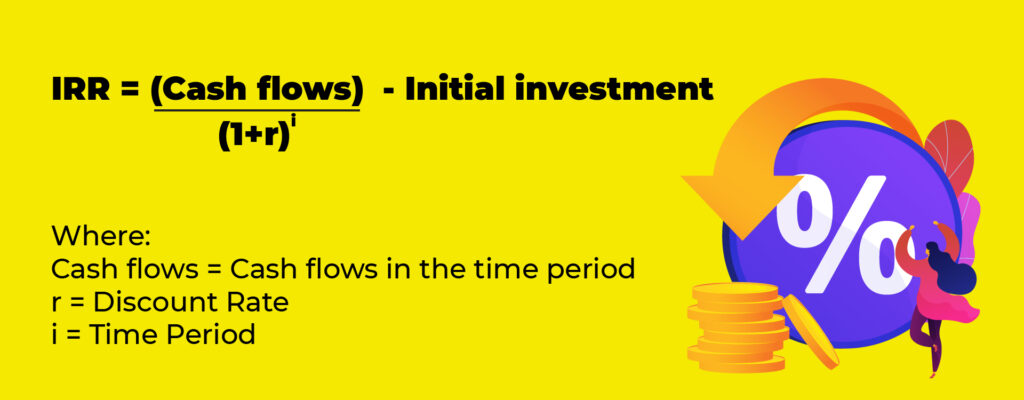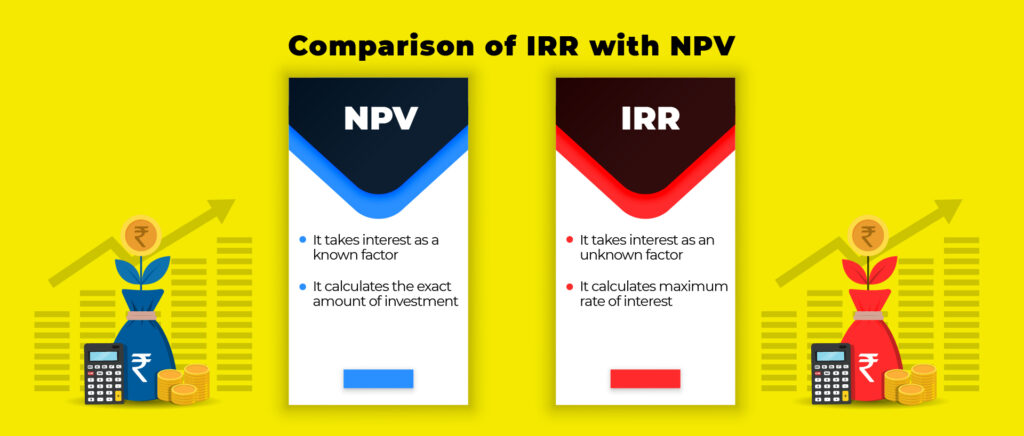# What is the Internal Rate of Return in Finance and Accounting

An in-depth understanding of Internal Rate of Return and how it is useful in the entrepreneurial landscape.

Internal Rate of Return has been used as a tool of profitability measurement for ages and has been recommended in various finance texts. Companies often discount the cash flows they receive before they try to calculate their payback period. They basically try to figure out how many years a project has to last so that it is advantageous when seen from the Net Present Value perspective. If the value of cash flows do not exceed the initial outlay, the discounted payback rule will reject the project. While if cash inflows exceed the initial outlay, long term projects continue to exist without the risk of rejection.

## Discounted Cash Flow or Internal Rate of Return

We always accept investment opportunities offering rates of return in excess of their opportunity costs of capital. The rate of return that generates a single payoff after one period is calculated by dividing the payoff with the investment and subtracting 1 from that value.

If we write Net Present Value (NPV) and try to find a discount rate that makes NPV=0, we find out that the discount rate that makes NPV=0 is nothing but the rate of return.

Now, this is for projects that generate payoffs in a single period. When we extend this concept to projects that produce cash flows in several periods, we get the project rate of return as the discount rate which gives zero NPV. This discount rate is known as the discounted cash flow rate of return or internal rate of return (IRR).

### We always accept investment opportunities offering rates of return in excess of their opportunity costs of capital.## Calculating IRR

The calculation of IRR is based on trial and error. Initially, we try a zero discount rate which gives us NPV positive. Since NPV is positive, IRR must be greater than zero. Then we try a discount rate of 50%, for instance if the NPV comes out to be negative. This implies that IRR is less than 50% and we keep doing this till we achieve NPV=zero at a particular discount rate. Usually, finance accounts plot graphs either manually or using computers to show the relationship between net present value and a range of discount rates. We finally figure out that at a discount rate of x%, the net present value is zero and thus the IRR is x%.

Most often, the most convenient way to calculate the IRR is to plot three or four combinations of NPV and discount rate on a graph and connect them to form a smooth line. Observing this graph, you can figure out the discount rate at which NPV is equal to zero. Nowadays, most companies use special programmes on computers and hire financial managers to conduct such calculations on a large scale.

## The Internal Rate of Return Rule

It is important to understand that IRR and opportunity cost of capital are very different measurement tools. IRR measures profitability and thus depends only on the amount and timing of project cash flows. While on the other hand, the opportunity cost of capital forms the standard of profitability which is used to calculate the worth of any project. It is the expected rate of return offered by other assets with the same risks as the project being evaluated.

The IRR rule states that we must accept an investment project if the opportunity cost of capital is less than the internal rate of return. At any discount rate, if the project has a positive NPV when discounted at the opportunity cost of capital, it implies that the opportunity cost of capital was less than the calculated IRR. If the opportunity cost is equal to the IRR, the project has zero NPV and if the opportunity cost exceeds IRR, then the project has a negative NPV. NPV is a smoothly declining function of discount rate which reflects whether or not the project has a positive NPV. The goal of IRR is to find out the rate of discount, which makes the present value of the sum of annual nominal cash inflows equal to the initial net cash outlay for the investment.

### Time Value of Money

The internal rate of return considers the time value of money when evaluating a project. One can measure IRR by calculating the interest rate at which the PV of future cash flows is equal to the capital investment required.

### Simplicity

IRR is very easy to calculate and interpret as compared to other measurements and is often used as the primary measure of project attractiveness. If the IRR exceeds the cost of capital, then accept the project, but not otherwise.

### Hurdle Rate Is Not Required

The hurdle rate is a difficult and subjective thing to decide. In IRR, there is no requirement to find out the IRR hurdle rate or the required rate of return. IRR does not depend on the hurdle rate thus reducing the false determination of the hurdle rate.## The Limitations of IRR

### Lending and Borrowing Pitfall

All cash-flow streams do not have NPV as a declining function of the discount rate. In some cases, we lend money at a discount rate by paying out while in other cases we receive or borrow money at some discount rate. When we lend money we want a higher discount rate while when we borrow money we want a low rate of return. In such cases where NPV increases with an increase in the discount rate, the IRR rule does not apply.

### More than one rate of return

Sometimes there are two discount rates at which the net present value is equal to zero. This happens when NPV initially increases with an increase in the discount rate and then declines with a further increase in the discount rate. There can be several internal rates of return for a project if there are changes in signs of the cash flows.

For instance, decommissioning and clean-up costs can be very large like removing offshore oil platforms may cost almost a billion dollars. These make cash flows go negative. Sometimes hotels need to get a facelift, machines may need repair or replacement, etc leading to negative cash flows. In such cases again, the IRR proves to be invalid.

### More than one opportunity cost of capital

When we discount, we discount the first payoff at the opportunity cost of capital for one year, the second payoff at the opportunity cost of capital for two years and so on and so forth. According to the IRR rule, we accept a project if the IRR is greater than the opportunity cost of capital. But this rule fails to apply when there are more than one opportunity costs. It leads to a lot of complexities in calculations leading to a failure in the calculation system.

### Mutually Exclusive Projects and IRR

Companies often have to choose between several alternative ways of doing the same job or using the same facilities and thus need to choose between mutually exclusive projects. Often it happens that suppose there are two projects X and Y, X involves a manually controlled machine while Y incorporates a higher level of technology in addition to labour. Both may seem like good investments but suppose X has a higher NPV compared to Y while Y has a higher IRR than X. Which do you prefer? Thus IRR fails to comply here. However, we can use IRR on incremental investment flows and check if that IRR is more than the opportunity cost. IRR is also unreliable in ranking projects offering different patterns of cash flow over time.Smruthi Krishnan
Smruthi interviews and writes on inspiring entrepreneurs and the success stories of start-ups. She is currently pursuing Economics major from Delhi University.

Disclaimer: The opinions expressed by columnists are their own, not those of Dutch Uncles

If you wish to contribute or have a story suggestion,
email to [email protected]